# 前言

25 法线贴图
33 曲面细分阶段(Tessellation)

1. 了解位移贴图
2. 熟悉如何用曲面细分来改善网格细节

DirectX11 With Windows SDK完整目录

Github项目源码

# 位移贴图（Displacement Mapping）

[mathbf{p’}=mathbf{p}+s(h-1)mathbf{n} ]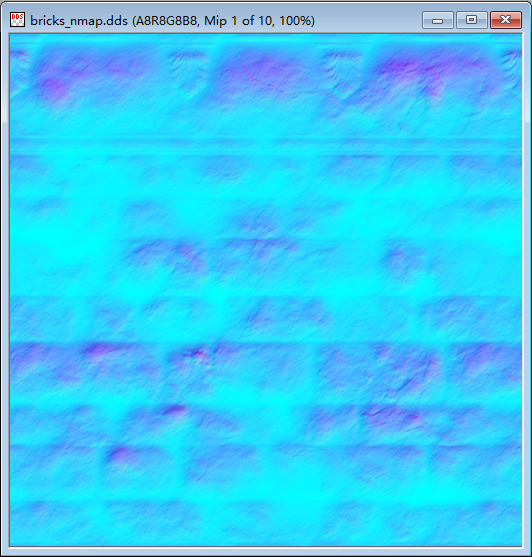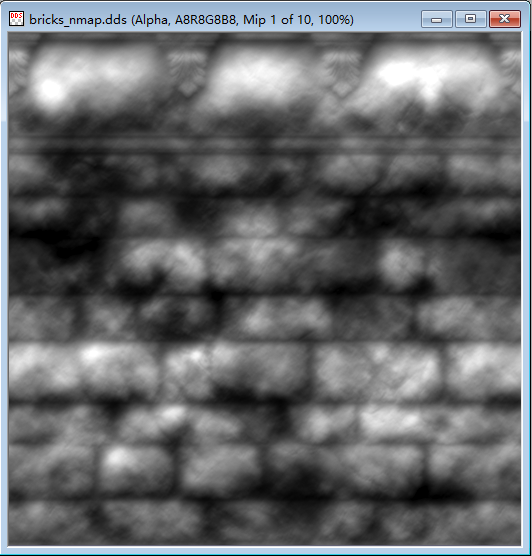# 位移贴图的着色器代码

## 顶点着色器

``cbuffer CBChangesEveryFrame {     // ...     float g_HeightScale;     float g_MaxTessDistance;     float g_MinTessDistance;     float g_MinTessFactor;     float g_MaxTessFactor; } ``
1. `g_MaxTessDistance`：从摄像机到该顶点的距离拉近到某个阈值时，将会达到最大的曲面细分因子
2. `g_MinTessDistance`：从摄像机到该顶点的距离拉远到某个阈值时，将会达到最小的曲面细分因子
3. `g_MinTessFactor`：曲面细分因子的最小值。比如说你想让每个三角形无论距离摄像机多远，都要让它最少被镶嵌成3份
4. `g_MaxTessFactor`：曲面细分因子的最大值。比如说你想让这些三角形无论距离摄像机多近，它最多的镶嵌份数不超过6.此外，回想起上一章所提到的建议，镶嵌后的三角形如果少于8个像素将会变得低效。

``// DisplacementMapObject_VS.hlsl #include "Basic.hlsli"  // 顶点着色器 TessVertexOut VS(VertexPosNormalTangentTex vIn) {     TessVertexOut vOut;      vOut.PosW = mul(float4(vIn.PosL, 1.0f), g_World).xyz;     vOut.NormalW = mul(vIn.NormalL, (float3x3) g_WorldInvTranspose);     vOut.TangentW = mul(vIn.TangentL, g_World);     vOut.Tex = vIn.Tex;          float d = distance(vOut.PosW, g_EyePosW);          // 标准化曲面细分因子     // TessFactor =      //   0, d >= g_MinTessDistance     //   (g_MinTessDistance - d) / (g_MinTessDistance - g_MaxTessDistance), g_MinTessDistance <= d <= g_MaxTessDistance     //   1, d <= g_MaxTessDistance     float tess = saturate((g_MinTessDistance - d) / (g_MinTessDistance - g_MaxTessDistance));          // [0, 1] --> [g_MinTessFactor, g_MaxTessFactor]     vOut.TessFactor = g_MinTessFactor + tess * (g_MaxTessFactor - g_MinTessFactor);          return vOut; }  ``
``// DisplacementMapInstance_VS.hlsl #include "Basic.hlsli"  // 顶点着色器 TessVertexOut VS(InstancePosNormalTangentTex vIn) {     TessVertexOut vOut;          vOut.PosW = mul(float4(vIn.PosL, 1.0f), vIn.World).xyz;     vOut.NormalW = mul(vIn.NormalL, (float3x3) vIn.WorldInvTranspose);     vOut.TangentW = mul(vIn.TangentL, vIn.World);     vOut.Tex = vIn.Tex;          float d = distance(vOut.PosW, g_EyePosW);          // 标准化曲面细分因子     // TessFactor =      //   0, d >= g_MinTessDistance     //   (g_MinTessDistance - d) / (g_MinTessDistance - g_MaxTessDistance), g_MinTessDistance <= d <= g_MaxTessDistance     //   1, d <= g_MaxTessDistance     float tess = saturate((g_MinTessDistance - d) / (g_MinTessDistance - g_MaxTessDistance));          // [0, 1] --> [g_MinTessFactor, g_MaxTessFactor]     vOut.TessFactor = g_MinTessFactor + tess * (g_MaxTessFactor - g_MinTessFactor);          return vOut; }   ``

## 外壳着色器

``PatchTess PatchHS(InputPatch<TessVertexOut, 3> patch,                   uint patchID : SV_PrimitiveID) {     PatchTess pt; 	     // 对每条边的曲面细分因子求平均值，并选择其中一条边的作为其内部的     // 曲面细分因子。基于边的属性来进行曲面细分因子的计算非常重要，这     // 样那些与多个三角形共享的边将会拥有相同的曲面细分因子，否则会导     // 致间隙的产生     pt.EdgeTess = 0.5f * (patch.TessFactor + patch.TessFactor);     pt.EdgeTess = 0.5f * (patch.TessFactor + patch.TessFactor);     pt.EdgeTess = 0.5f * (patch.TessFactor + patch.TessFactor);     pt.InsideTess = pt.EdgeTess; 	     return pt; } ``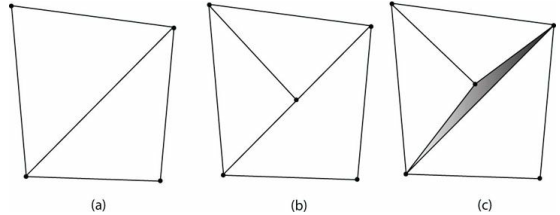``// 外壳着色器 [domain("tri")] [partitioning("fractional_odd")] [outputtopology("triangle_cw")] [outputcontrolpoints(3)] [patchconstantfunc("PatchHS")] HullOut HS(InputPatch<TessVertexOut, 3> patch,     uint i : SV_OutputControlPointID,     uint patchId : SV_PrimitiveID) {     HullOut hOut; 	 	// 直传     hOut.PosW = patch[i].PosW;     hOut.NormalW = patch[i].NormalW;     hOut.TangentW = patch[i].TangentW;     hOut.Tex = patch[i].Tex; 	     return hOut; }  ``

## 域着色器

``// DisplacementMap_DS.hlsl #include "Basic.hlsli"  [domain("tri")] VertexOutNormalMap DS(PatchTess patchTess,              float3 bary : SV_DomainLocation,              const OutputPatch<HullOut, 3> tri) {     VertexOutNormalMap dOut;          // 对面片属性进行插值以生成顶点     dOut.PosW     = bary.x * tri.PosW     + bary.y * tri.PosW     + bary.z * tri.PosW;     dOut.NormalW  = bary.x * tri.NormalW  + bary.y * tri.NormalW  + bary.z * tri.NormalW;     dOut.TangentW = bary.x * tri.TangentW + bary.y * tri.TangentW + bary.z * tri.TangentW;     dOut.Tex      = bary.x * tri.Tex      + bary.y * tri.Tex      + bary.z * tri.Tex;          // 对插值后的法向量进行标准化     dOut.NormalW = normalize(dOut.NormalW);          //     // 位移映射     //          // 基于摄像机到顶点的距离选取mipmap等级；特别地，对每个MipInterval单位选择下一个mipLevel     // 然后将mipLevel限制在[0, 6]     const float MipInterval = 20.0f;     float mipLevel = clamp((distance(dOut.PosW, g_EyePosW) - MipInterval) / MipInterval, 0.0f, 6.0f);          // 对高度图采样（存在法线贴图的alpha通道）     float h = g_NormalMap.SampleLevel(g_Sam, dOut.Tex, mipLevel).a;          // 沿着法向量进行偏移     dOut.PosW += (g_HeightScale * (h - 1.0f)) * dOut.NormalW;          // 生成投影纹理坐标     dOut.ShadowPosH = mul(float4(dOut.PosW, 1.0f), g_ShadowTransform);          // 投影到齐次裁减空间     dOut.PosH = mul(float4(dOut.PosW, 1.0f), g_ViewProj);          // 从NDC坐标[-1, 1]^2变换到纹理空间坐标[0, 1]^2     // u = 0.5x + 0.5     // v = -0.5y + 0.5     // ((xw, yw, zw, w) + (w, w, 0, 0)) * (0.5, -0.5, 1, 1) = ((0.5x + 0.5)w, (-0.5y + 0.5)w, zw, w)     //                                                      = (uw, vw, zw, w)     //                                                      =>  (u, v, z, 1)     dOut.SSAOPosH = (dOut.PosH + float4(dOut.PosH.ww, 0.0f, 0.0f)) * float4(0.5f, -0.5f, 1.0f, 1.0f);          return dOut; }  ``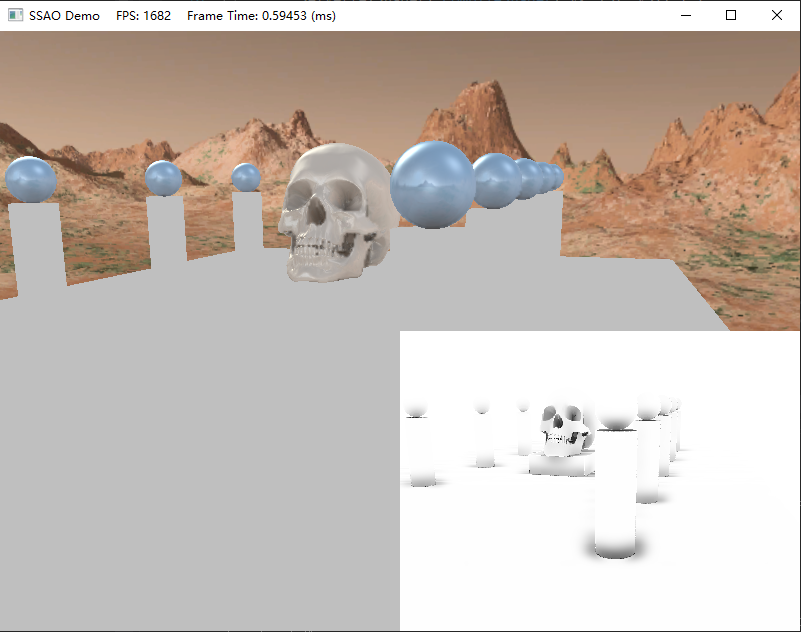`DisplacementMapObject_VS` `SSAO_NormalDepth_ObjectTess_VS` `ShadowObjectTess_VS`
`DisplacementMapInstance_VS` `SSAO_NormalDepth_InstanceTess_VS` `ShadowInstanceTess_VS`
`DisplacementMap_HS` `SSAO_NormalDepth_HS` `Shadow_DS`
`DisplacementMap_DS` `SSAO_NormalDepth_DS` `Shadow_HS`

# 演示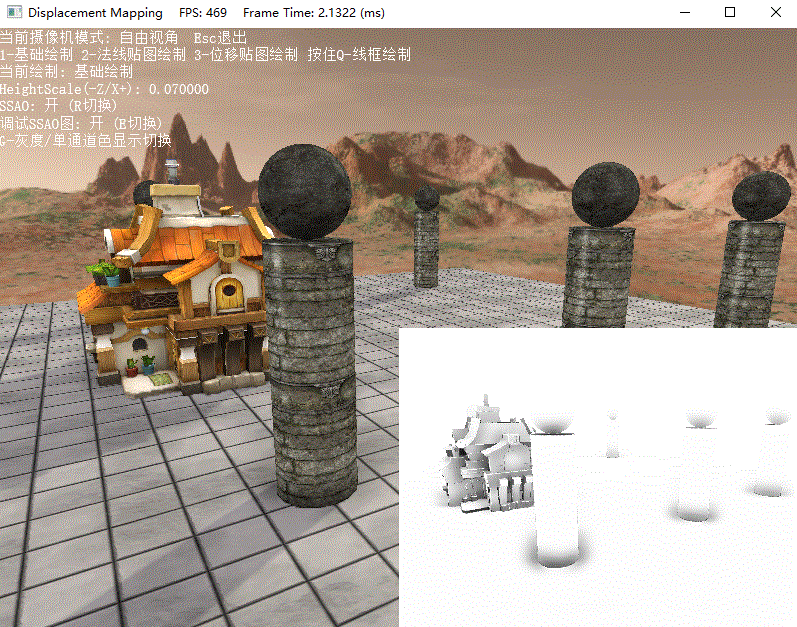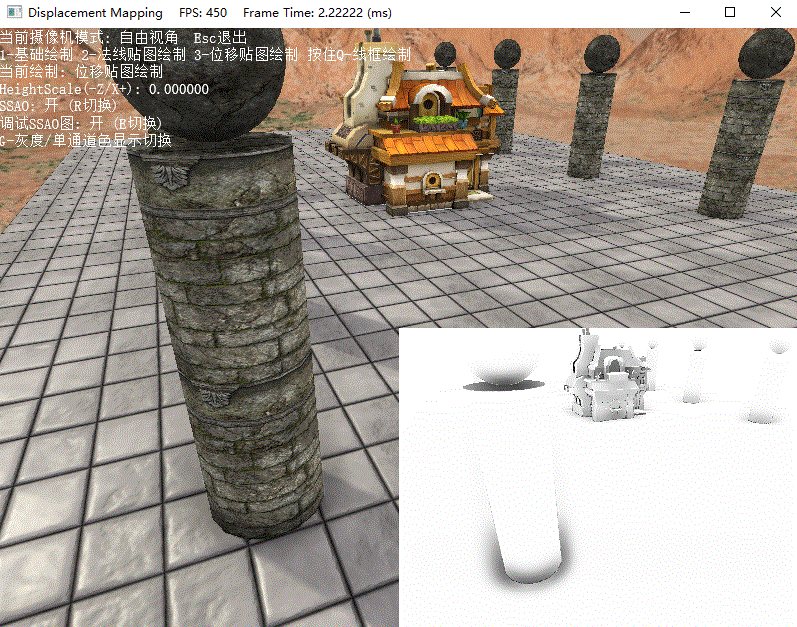DirectX11 With Windows SDK完整目录

Github项目源码

X_Jun资料部分资料来自网络，侵权毕设源码联系删除

qklbishe.com区块链毕设代做网专注|以太坊fabric-计算机|java|毕业设计|代做平台 » X_Jun资料